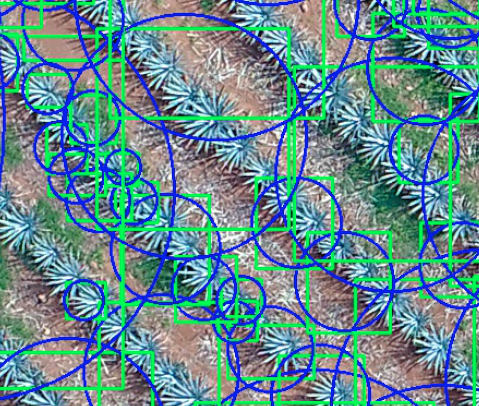# How to count images

How can I count the agave plants in this field? what technique do you recommend??

thanks

You could start with a naive approach of trying to predict the target value directly or alternatively use an object detection task, where you could count the predicted bounding boxes for each plant.as you see not being able to identify only the plants:
my code is

``````
#conversion a escala de grises
gray=cv2.cvtColor(img,cv2.COLOR_BGR2GRAY)
gray = cv2.GaussianBlur(gray,(25,25),0)

ret, threshed_img = cv2.threshold(cv2.cvtColor(img, cv2.COLOR_BGR2GRAY),
127, 255, cv2.THRESH_BINARY)
# find contours and get the external one

dilated = cv2.dilate(thresh,kernel,iterations = 13) # dilate
contours, hierarchy = cv2.findContours(dilated,cv2.RETR_EXTERNAL,cv2.CHAIN_APPROX_NONE)

for cnt in contours:
x,y,w,h = cv2.boundingRect(cnt)
if h<30 or w<30:
continue
else:

cv2.rectangle(img, (x, y), (x+w, y+h), (0, 255, 0), 2)
# get the min area rect
rect = cv2.minAreaRect(cnt)
box = cv2.boxPoints(rect)
# convert all coordinates floating point values to int
box = np.int0(box)
# draw a red 'nghien' rectangle
#cv2.drawContours(img, [box], 0, (0, 0, 255))

# finally, get the min enclosing circle
(x, y), radius = cv2.minEnclosingCircle(cnt)
# convert all values to int
center = (int(x), int(y))
If you don’t have the labels and would like to use a classical computer vision approach, I would recommend to post the question in an OpenCV-specific discussion board or on StackOverflow, as your use case doesn’t seem to be PyTorch-specific.